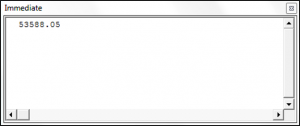# VBA Timer Function

The VBA Timer functions returns the number of seconds that have elapsed since midnight of the current day (returned as a Single data type).

## Using the VBA Timer Function

The following code will show you how to use the VBA Timer Function to determine how many seconds have passed, since midnight, at the time the code is being run:

``````Sub UsingTheVBATimerFunction()

Dim secondsSince As Single

secondsSince = Timer()
Debug.Print secondsSince

End Sub``````

The result is:## Use VBA Timer to get Actual Time

You can convert the seconds returned from the Timer function into hh:mm:ss format to see the actual time by using the following code:

``````Sub GettingTheActualTime()

Dim secondsSince As Single
Dim cTime As Double
Dim theActualTime As Variant

secondsSince = Timer()

cTime = secondsSince / (86400)
theActualTime = Format(cTime, "hh:mm:ss")

MsgBox "The time elapsed since midnight in seconds is" & " " & secondsSince & vbNewLine & _
"The actual time is:" & " " & theActualTime

End Sub``````

The result is:## Time a Section of VBA Code

If you are looking to benchmark re-written code or debate “quicker” methods in VBA you can use VBA’s built-in timer. Setting a variable equal to the timer at the start of your code and subtracting this from the timer at the end will give you a good estimate of how long a piece of code takes to run.

Performance may be affected by other programs running or trying to run while you’re macro is active, among other things.

The following example was used to see how long it would take to write the word “test” to cell A1 on Sheet1 a half million times. It took 21 seconds on my machine.

``````Sub BenchMark()

Dim Count As Long
Dim BenchMark As Double

BenchMark = Timer

'Start of Code to Test

For Count = 1 To 500000
Sheet1.Cells(1, 1) = "test"
Next Count

'End of Code to Test

MsgBox Timer - BenchMark

End Sub``````

If your code is running slowly, try speeding it up by disabling screen updating.   To keep the Excel screen active while the timer is running, we can insert the DoEvents method into the code.

Stop searching for VBA code online. Learn more about AutoMacro - A VBA Code Builder that allows beginners to code procedures from scratch with minimal coding knowledge and with many time-saving features for all users!